1. /
2. CBSE
3. /
4. Class 08
5. /
6. Mathematics
7. /
8. NCERT Solutions for Class...

# NCERT Solutions for Class 8 Maths Exercise 14.4### myCBSEguide App

Download the app to get CBSE Sample Papers 2023-24, NCERT Solutions (Revised), Most Important Questions, Previous Year Question Bank, Mock Tests, and Detailed Notes.

NCERT solutions for Class 8 Maths Factorisation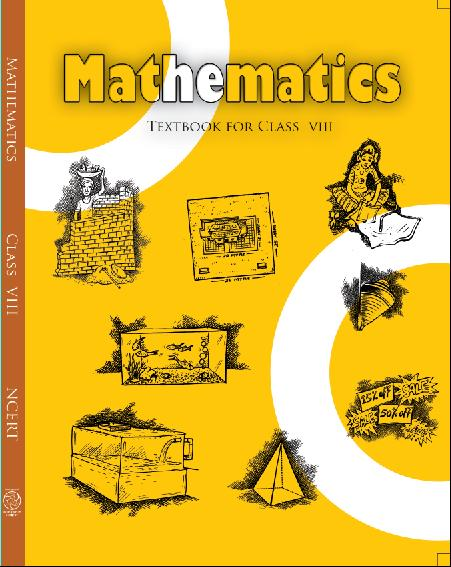## NCERT Solutions for Class 8 Maths Factorisation

Class –VIII Mathematics(Ex. 14.4)

NCERT SOLUTION

Find and correct the errors in the following mathematical statements:

1.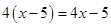Ans. L.H.S. =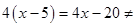R.H.S.

Hence the correct mathematical statements is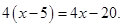2.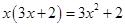Ans. L.H.S. =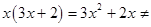R.H.S.

Hence the correct mathematical statements is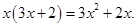3.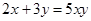Ans. L.H.S. =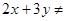R.H.S.

Hence the correct mathematical statements is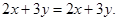4.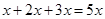Ans. L.H.S. =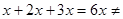R.H.S.

Hence the correct mathematical statements is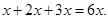5.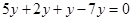Ans. L.H.S. =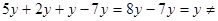R.H.S.

Hence the correct mathematical statements is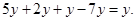6.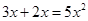Ans. L.H.S. =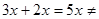R.H.S.

Hence the correct mathematical statements is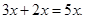7.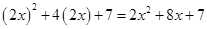Ans. L.H.S. =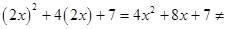R.H.S.

Hence the correct mathematical statements is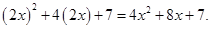8.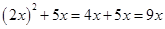Ans. L.H.S. =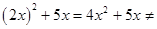R.H.S.

Hence the correct mathematical statements is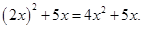9.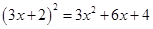Ans.

L.H.S. =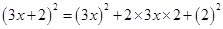.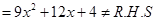Hence the correct mathematical statements is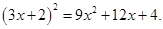10. Substituting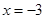in:

(a)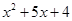gives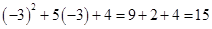(b)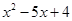gives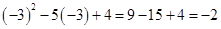(c)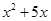gives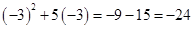Ans. (a) L.H.S. =Puttingin given expression,

=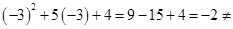R.H.S.

Hencegives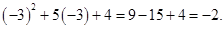(b) L.H.S. =Puttingin given expression,

=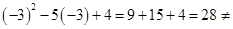R.H.S.

Hencegives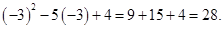(c) L.H.S. =Puttingin given expression,

=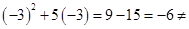R.H.S.

Hencegives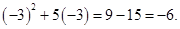11.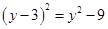Ans. L.H.S. =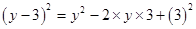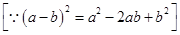=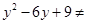R.H.S.

Hence the correct statements is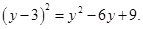12.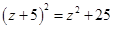Ans. L.H.S. =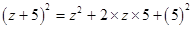=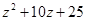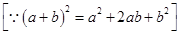Hence the correct statement is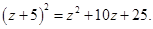13.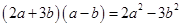Ans. L.H.S. =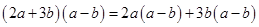=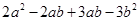=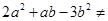R.H.S.

Hence the correct statement is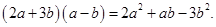14.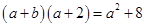Ans. L.H.S. =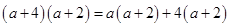=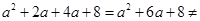R.H.S.

Hence the correct statement is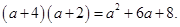15.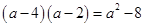Ans. L.H.S. =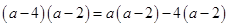=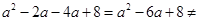R.H.S.

Hence the correct statement is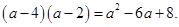16.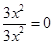Ans. L.H.S. =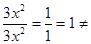R.H.S.

Hence the correct statement is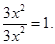17.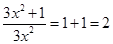Ans. L.H.S. =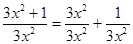=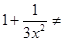R.H.S.

Hence the correct statement is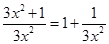.

18.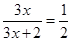Ans. L.H.S. =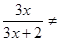R.H.S.

Hence the correct statement is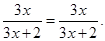NCERT Solutions for Class 8 Maths Exercise 14.4

19.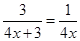Ans. L.H.S. =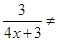R.H.S.

Hence the correct statement is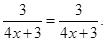20.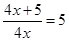Ans. L.H.S. =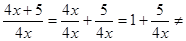R.H.S.

Hence the correct statement is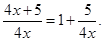NCERT Solutions for Class 8 Maths Exercise 14.4

21.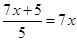Ans. L.H.S. =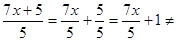R.H.S.

Hence the correct statement is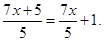## NCERT Solutions for Class 8 Maths Exercise 14.4

NCERT Solutions Class 8 Mathematics PDF (Download) Free from myCBSEguide app and myCBSEguide website. Ncert solution class 8 Mathematics includes text book solutions from Class 8 Maths Book . NCERT Solutions for CBSE Class 8 Maths have total 16 chapters. 8 Maths NCERT Solutions in PDF for free Download on our website. Ncert class 8 solutions PDF and Maths ncert class 8 PDF solutions with latest modifications and as per the latest CBSE syllabus are only available in myCBSEguide.

## CBSE app for Class 8

To download NCERT Solutions for class 8 Social Science, Computer Science, Home Science,Hindi ,English, Maths Science do check myCBSEguide app or website. myCBSEguide provides sample papers with solution, test papers for chapter-wise practice, NCERT solutions, NCERT Exemplar solutions, quick revision notes for ready reference, CBSE guess papers and CBSE important question papers. Sample Paper all are made available through the best app for CBSE students and myCBSEguide website.Test Generator

Create question paper PDF and online tests with your own name & logo in minutes.myCBSEguide

Question Bank, Mock Tests, Exam Papers, NCERT Solutions, Sample Papers, Notes

### 30 thoughts on “NCERT Solutions for Class 8 Maths Exercise 14.4”

1. Not good . they have not done briefly very small answers

2. Very good sight

3. Very Nice

4. Thank you so muchhhhhh!

5. Nice

6. Very good

7. Thank you ?

8. Very good site

9. Thanks for the answer it makes my notebook complete thank u so much

10. Thank you

11. thanks..

12. Helped a lotttttt.

13. Good

14. Very very useful.
Thanks

15. Very very useful.
Thanks.

16. Thanks, it helped me a lot. It is a good site

17. It’s helpful to my children

18. Good

Best

I like to copy and paste on day before submission

20. I THINK IS NICE

22. It help me a lot .
Thanks for helping me.

23. sooper it was very usefull

24. Seriously, it helped a lot nd I understood it easily.
Thanku so much.

25. Thank,s it my help

26. It is very important to me that you will be able to help me with the following details of my work and I will be grateful to help you to following benefits to help you to achieve

27. It is very good

28. It is very useful ?????

29. It is very useful ?????

30. It is good?